# Ornstein isomorphism theorem

Ergodic theory, the study of measure-preserving transformations or flows, arose from the study of the long-term statistical behaviour of dynamical systems (cf. also Measure-preserving transformation; Flow (continuous-time dynamical system); Dynamical system). Consider, for example, a billiard ball moving at constant speed on a rectangular table with a convex obstacle. The state of the system (the position and velocity of the ball), at one instant of time, can be described by three numbers or a point in Euclidean 3-dimensional space, and its time evolution by a flow on its state space, a subset of 3-dimensional space. The Lebesgue measure of a set does not change as it evolves and can be identified with its probability.

One can abstract the statistical properties (e.g., ignoring sets of probability 0) and regard the state-space as an abstract measure space. Equivalently, one says that two flows are isomorphic if there is a one-to-one measure-preserving (probability-preserving) correspondence between their state spaces so that corresponding sets evolve in the same way (i.e., the correspondence is maintained for all time).

It is sometimes convenient to discretize time (e.g., look at the flow once every minute), and this is also referred to as a transformation.

Measure-preserving transformations (or flows) also arise from the study of stationary processes (cf. also Stationary stochastic process). The simplest examples are independent processes such as coin tossing. The outcome of each coin tossing experiment (the experiment goes on for all time) can be described as a doubly-infinite sequence of heads $$H$$ and tails $$T$$. The state space is the collection of these sequences. Each subset is assigned a probability. For example, the set of all sequences that are $$H$$ at time 3 and $$T$$ at time 5 gets probability 1/4. The passage of time shifts each sequence to the left (what used to be time 1 is now time 0). (This kind of construction works for all stochastic processes, independence and discrete time are not needed.)

The above transformation is called the Bernoulli shift $$B(1/2,1/2)$$. If, instead of flipping a coin, one spins a roulette wheel with three slots of probability $$p_1, p_2, p_3$$, one would get the Bernoulli shift $$B(p_1, p_2, p_3)$$.

Bernoulli shifts play a central role in ergodic theory, but it was not known until 1958 whether or not all Bernoulli shifts are isomorphic. A.N. Kolmogorov and Ya.G. Sinai solved this problem by introducing a new invariant for measure-preserving transformations: the entropy, which they took from Shannon's theory of information (cf. also Entropy of a measurable decomposition; Shannon sampling theorem). They showed that the entropy of $$B(p_1,\ldots,p_n)$$ is

$\sum_{i=1}^n p_i \log p_i$

thus proving that not all Bernoulli shifts are isomorphic.

The simplest case of the Ornstein isomorphism theorem (1970), [a3], states that two Bernoulli shifts of the same entropy are isomorphic.

A deeper version says that all the Bernoulli shifts are strung together in a unique flow: There is a flow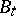such thatis isomorphic to the Bernoulli shift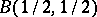, and for any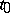,is also a Bernoulli shift. (Here,means that one samples the flow everyunits of time.) In fact, one obtains all Bernoulli shifts (more precisely, all finite entropy shifts) by varying. (There is also a unique Bernoulli flow of infinite entropy.)is unique up to a constant scaling of the time parameter (i.e., if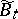is another flow such that for some,is a Bernoulli shift, then there is a constantsuch that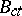is isomorphic to).

The thrust of this result is that at the level of abstraction of isomorphism there is a unique flow that is the most random possible.

The above claim is clarified by the following part of the isomorphism theorem: Any flowthat is not completely predictable, has as a factorfor some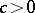(the numbersinvolved are those for which the entropy ofis not greater than the entropy of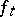). The only factors ofarewith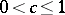.

Here, completely predictable means that all observations on the system are predictable in the sense that if one makes the observation at regular intervals of time (i.e., every hour on the hour), then the past determines the future. (An observation is simply a measurable functionon the state space; one can think of repeated observations as a stationary process.) It is not hard to prove that "completely predictable" is the same as zero entropy.

Also, "Bt is a factor of ft" means that there is a many-to-one mappingfrom the state space ofto that ofso that a set and its inverse image evolve in the same way (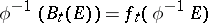; this is the same as saying that one getsby restrictingto an invariant sub-sigma algebra or by lumping points).

Thus,is, in some sense, responsible for all randomness in flows.

The most important part of the isomorphism theorem is a criterion that allows one to show that specific systems are isomorphic to.

Using results of Sinai, one can show that billiards with a convex obstacle (described earlier) are isomorphic to.

If one would perturb the obstacle, one would get an isomorphic system; if the perturbation is small, then the isomorphism mapping of the state space (a subset of-dimensional space) can be shown to be close to the identity. This is an example of "statistical stability" , another consequence of the isomorphism theorem, which provides a statistical version of structural stability. Note that the billiard system is very sensitive to initial conditions and the perturbation completely changes individual orbits. This result shows, however, that the collection of all orbits is hardly changed.

Geodesic flow on a manifold of negative curvature is another example where results of D. Anosov allow one to check the criterion and is thus isomorphic to. Here too one obtains stability for small perturbations of the manifold's Riemannian structure.

Results of Ya.B. Pesin allow one to check the criterion for any ergodic measure preserving flow of positive entropy on a-dimensional manifold (i.e. not completely predictable). Thus, any such flow is isomorphic toor the product ofand a rotation. Stability is not known.

How to Cite This Entry:
Ornstein isomorphism theorem. Encyclopedia of Mathematics. URL: http://encyclopediaofmath.org/index.php?title=Ornstein_isomorphism_theorem&oldid=27176
This article was adapted from an original article by D. Ornstein (originator), which appeared in Encyclopedia of Mathematics - ISBN 1402006098. See original article